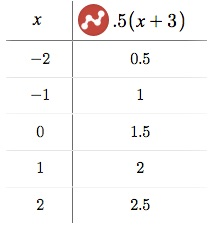# POLQ 3 | Lesson 1 | Explore (Multiplying two Linear Functions) Example

The original equation was $$y = \dfrac{1}{2}(x+3)$$

another equation $$y = 2(x-2)$$

Table of values:Notice that in the first two equations $$-c$$ appears to be the $$x$$ intercept. That is the red line and the blue line above.  When multiplied together the equation becomes a quadratic.

Tables and graphs are created using Desmos.com.